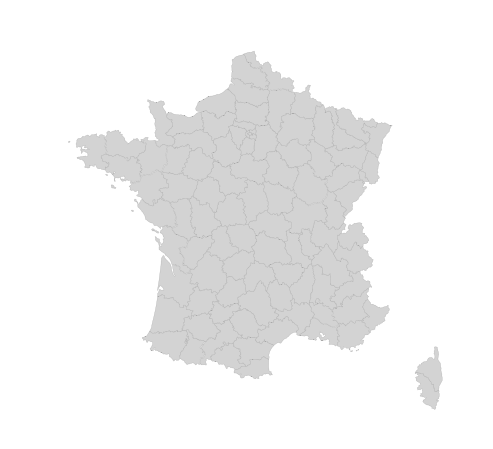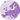# Draw a map from a geojson file with Python, GeoPandas and GeoPlot

This post explains how to load a `geoJson` file with python and transform it into a `GeoDataFrame` with `GeoPandas`. Once this `GeoDataFrame` is available, it is ready to be manipulated and plotted with a library like `Geoplot` as shown below 🔥!

## 🕘 Quick start

You have a `geoJson` file and want to draw a map with it:

## 🤔 What is a `geoJson` file?

This is a geoJson file. Let's consider french state boundaries. The original file comes fromo here but I stored it on the github repo of the python graph gallery for convenience.

``````%%html

## How to load a `geoJson` file into python

You're best friend here is `geopandas`. If this package hasn't been installed on your machine yet, you can do so with `pip install geopandas`. Then, load the package:

Note to self: this page is helpful if you're struggling to install `geopandas` on a Mac. This one as well.

``import geopandas as gpd``
``gpd.datasets.available``
``['naturalearth_cities', 'naturalearth_lowres', 'nybb']``

Now, let's load the `.geojson` file located here.

``data = gpd.read_file("https://raw.githubusercontent.com/holtzy/The-Python-Graph-Gallery/master/static/data/france.geojson")``
``data.crs``
``````<Geographic 2D CRS: EPSG:4326>
Name: WGS 84
Axis Info [ellipsoidal]:
- Lat[north]: Geodetic latitude (degree)
- Lon[east]: Geodetic longitude (degree)
Area of Use:
- name: World
- bounds: (-180.0, -90.0, 180.0, 90.0)
Datum: World Geodetic System 1984
- Ellipsoid: WGS 84
- Prime Meridian: Greenwich``````

Here we are 🎉! `data` is a `geo dataframe`. Each row represents an item in the `geojson` file, i.e. a region of france here. Columns describe the feature of each region. The `geometry` column is probably the most important. It provides the coordinate of the region boundary.

``print(type(data))``
```<class 'geopandas.geodataframe.GeoDataFrame'> ```

%%html

``````#import requests
#import pandas as pd
#url = 'https://github.com/holtzy/The-Python-Graph-Gallery/blob/master/static/data/france.geojson'
#response = requests.get(url)
#response
#data = response.json()
#data
##df = pd.io.json.json_normalize(data['features'])
#df``````

Many different options exist to plot a map from a geopandas dataframe. The most common solution is to use a package called `geoplot`. You can install it with `conda` with `conda install geoplot -c conda-forge`. (See here). Then import it with:

``````import geoplot
import geoplot.crs as gcrs``````

Once the library is loaded, the `polyplot()` function can be used to draw a map of the geospatial data frame. The `polyplot()` function is used to plot polygons, i.e any type of geographic area.

``````geoplot.polyplot(data, projection=gcrs.AlbersEqualArea(), edgecolor='darkgrey', facecolor='lightgrey', linewidth=.3,
figsize=(12, 8))``````
``<GeoAxesSubplot:>``Here we are, we've loaded a `geoJson` file, transformed it into a `geopandas dataframe` and drawn a map with `geoplot` from it!

## Contact & Edit

👋 This document is a work by Yan Holtz. Any feedback is highly encouraged. You can fill an issue on Github, drop me a message onTwitter, or send an email pasting `yan.holtz.data` with `gmail.com`.